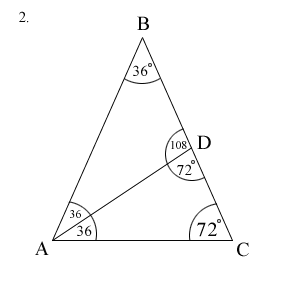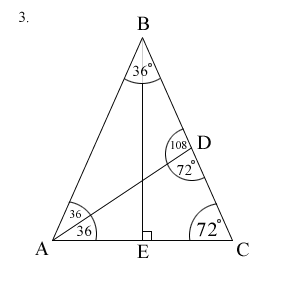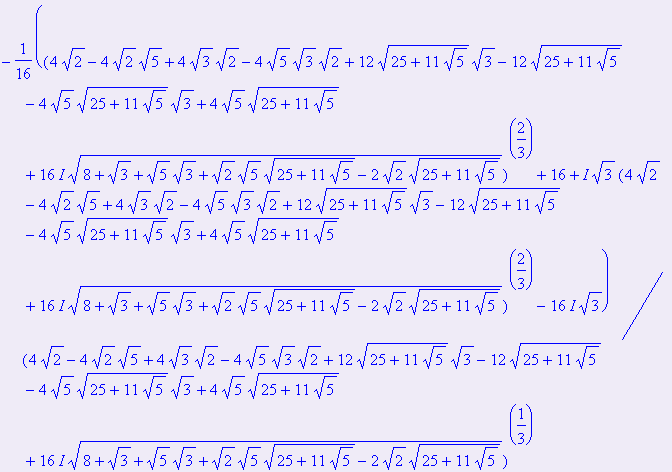# Finding the Sine of 1 Degree

Is it possible to find an exact radical expression for sin(1º)? Yes. The approach that will be taken is similar to a common set of techniques used when attempting to determine the sine of an angle using previous knowledge on the sine of a larger (or smaller) angle.

Looking at commonly known values of sin(x): sin(60º), sin(45º), sin(30º), a somewhat reasonable attempt at finding sin(1º) (or [for now] a value of the sine of an integral value close to 1º) would be by using these values along with well-known identities (and using any values of sin(x) that were derived in the process).

For instance:

 (1) sin(15º) = sin(45º - 30º) By sum identity (2) sin(15º/2) = sin(7.5º) By half-angle identity (3) sin(45º/2) = sin(22.5º) " " " " (4) sin(22.5º/2) = sin(11.25º) " " " " (5) sin(7.5º) = sin(11.25º - 3.75º) By sum identity (6) sin(11.25º/2) = sin(5.625º) By half-angle identity (7) sin(5.625º - 3.75º) = sin(1.875º) By sum identity

## Using Other Values of sin(x)

Unfortunately, it seems fruitless (or extremely difficult) to generate a value of sin(1º) (or the sine of an integral value very close to one) by solely manipulating the values in the list above. However, there is an angle that can be used with the commonly known values of sin(x) that will take us an extremely significant step closer to sin(1º). 72º. Before making use of sin(72º), we must be able to find it. A geometric approach for finding the sine of 72º is shown below:Given a triangle ABC, impose a line segment AD on angle A such that AD bisects angle A and D lies on BC.There are similarities generated among the sides of triangles ABC and ADC. Note that BC / AC = AD / DC. Since our intermediate goal is to find sin(72º), a ratio, we are free to let DC = 1. Then, let BD = x. This leads to AD = x and AC = x which leads to: (x + 1) / x = x / 1, x^2 - x - 1 = 0. Solving the quadratic for its positive value, x = (1/2)(1 + sqrt(5)). Note this value for the steps to follow.

Next, erect a perpendicular from a point E on AC which extends to angle B.Now we can define sin(72º) = BE / BC. Recall BC = x + 1. Note (BE)^2 + (EC)^2 = (BC)^2 and since EC = AC / 2, this implies BE = sqrt((BC)^2 - (AC / 2)^2). Since AC = x, BE = sqrt((x + 1)^2 - (x / 2)^2). Plugging in for x, BE = sqrt((25 + 11sqrt(5)) / 8). This leads to sin(72º) = sqrt((25 + 11sqrt(5)) / 8) / ((3 + sqrt(5) / 2) which somewhat simplifies to sqrt(25 + 11sqrt(5))/(3sqrt(2) + sqrt(10)). cos(72º) can be similarly obtained from the triangle. cos(72º) = (sqrt(5) - 1) / 4.

## Going Back

Now that sin(72º) is known. We can go back and try once again to find sin(1º) (or as before, the sine of a close integral value to 1º) by various manipulations of other known values of sin(x).

 (1) sin(75º) = sin(45º + 30º) By sum identity (2) sin(3º) = sin(75º - 72º) By sum identity

Having the value of sin(3º) will prove useful. Let us compute it.

sin(3º) = sin(75º - 72º) = sin(75º)cos(72º) - cos(75º)sin(72º)

sin(75º) = (sqrt(6) + sqrt(2)) / 4, cos(75º) = (sqrt(6) - sqrt(2)) / 4

sin(3º) = [(sqrt(6) + sqrt(2)) / 4] * [(sqrt(5) - 1) / 4] - [(sqrt(6) - sqrt(2)) / 4] * [sqrt(25 + 11sqrt(5))/(3sqrt(2) + sqrt(10))]

## Reduced to Solving a Cubic

Is it possible to express sin(1º) in terms of sin(3º)? Certainly.

Using a third-angle formula sin(3x) = 3sin(x) - 4sin^3(x), let x = 1º. Then:

sin(3º) = 3sin(1º) - 4sin^3(1º)

Let z = sin(1º), this leads to 4z^3 - 3z + sin(3º) = 0.

Fortunately, the exact solutions can be found with the cubic formula. Note that the numerical approximations of the solutions are -.8746197071, .01745240644, and .8571673007. Simply by looking at sin(30º) = 1/2 and the fact that sin(a) > sin(b) for a,b in [0,90º] implies a > b we see .8571673007 = sin(c), for some c > 30º which eliminates the third solution. The first solution is disregarded since sin(x) => 0 for x in [0,90º] leaving us with the second solution as an approximation to sin(1º).

Using Maple or Mathematica, one can readily find z. Here is a capture of the exact expression that approximates to the second solution of the cubic. This was shown via Maple. (sin(3º) is written as sin(pi / 60):Substituting the radical value of sin(3º) yields the colossal expression below. Therefore, sin(1º) =You may have noticed some of the terms contain factors of i. However, the imaginary part of the expression equates to zero. Thus, it is of the real form a + 0i as we would expect for any value of sin(x) for x in R.

Questions? Comments? sk@ull.edu or /msg Meta in #math

EFnet #math Home